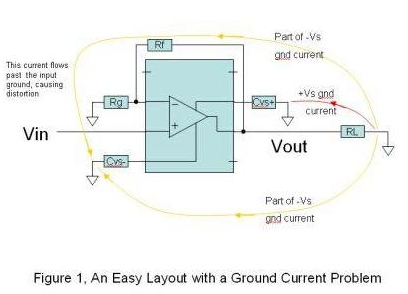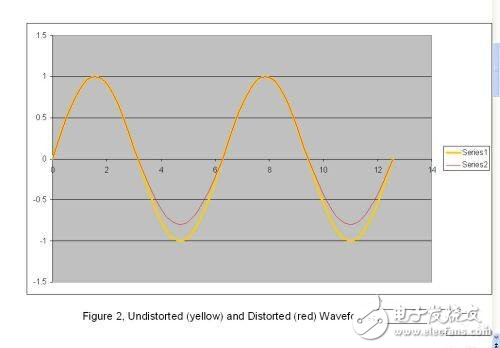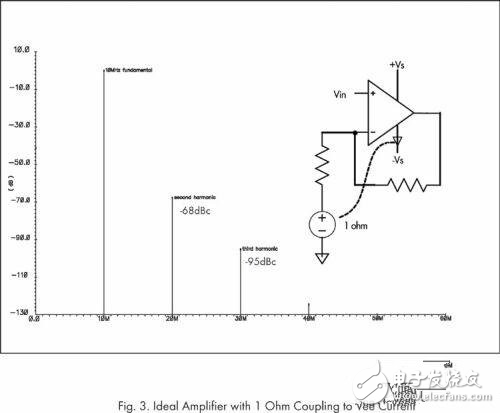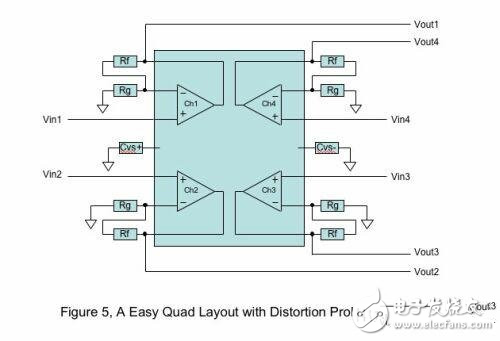# How to avoid distortion when designing high frequency PCB layout

In fact, printed circuit boards (PCBs) are made of electrically linear materials, ie their impedance should be constant. So why does the PCB introduce nonlinearity into the signal? The answer is that the PCB layout is “space-non-linear” relative to where the current flows.

Whether the amplifier draws current from this source or from another source depends on the instantaneous polarity of the signal on the applied load. Current flows from the power supply, through the bypass capacitor, through the amplifier into the load. The current then returns from the load ground (or the shield of the PCB output connector) to the ground plane, through the bypass capacitor, back to the power supply that originally supplied the current.

The concept of current flowing through the minimum path of impedance is incorrect. The amount of current in all different impedance paths is proportional to its conductivity. At a ground level, there is often a low-impedance path through which a large proportion of current flows: one path is directly connected to the bypass capacitor; the other forms an excitation of the input resistance before reaching the bypass capacitor. Figure 1 illustrates these two paths. Ground return current is the real cause of the problem.When the bypass capacitors are placed at different locations on the PCB, the ground current flows through the different paths to the respective bypass capacitors, meaning "space nonlinearity". If a large portion of the component of a certain polarity of the ground current flows through the ground of the input circuit, only the component voltage of this polarity of the signal is disturbed. If the other polarity of the current is not disturbed, the input signal voltage changes in a non-linear manner. When one polarity component changes and the other polarity does not change, distortion occurs and appears as the second harmonic distortion of the output signal. Figure 2 shows this distortion effect in an exaggerated form.When only one polar component of a sine wave is disturbed, the resulting waveform is no longer a sine wave. Simulating an ideal amplifier with a 100Ω load causes the load current to pass through a 1Ω resistor, coupling the input ground voltage only to one polarity of the signal, resulting in the results shown in Figure 3. The Fourier transform shows that the distortion waveform is almost entirely the second harmonic at -68dBc. When the frequency is high, it is easy to generate this degree of coupling on the PCB, which can destroy the excellent anti-aliasing characteristics of the amplifier without much special nonlinear effects of the PCB. When the output of a single op amp is distorted due to the ground current path, the current is regulated by rearranging the bypass loop and maintaining the distance from the input device, as shown in Figure 4.Multi-amplifier chip

The problem with multi-amplifier chips (two, three or four amplifiers) is more complicated because it does not allow the ground connection of the bypass capacitors to be kept away from all inputs. This is especially true for four amplifiers. Each side of the quad amplifier chip has an input, so there is no space to place a bypass circuit that mitigates disturbances to the input channel.

Figure 5 shows an easy way to layout a four-amplifier. Most devices are directly connected to the four amplifier pins. The ground current of one power supply can disturb the input ground voltage and ground current of the other channel power supply, resulting in distortion. For example, the (+Vs) bypass capacitor on channel 4 of the four amplifiers can be placed directly adjacent to its input; the (-Vs) bypass capacitor can be placed on the other side of the package. (+Vs) ground current can disturb channel 1, while (-Vs) ground current may not.

To avoid this problem, ground current can be disturbed by the input, but the PCB current flows in a spatially linear manner. To achieve this, the bypass capacitor can be placed on the PCB in the following way: currents of (+Vs) and (–Vs) flow through the same path. If the positive/negative currents are equal to the input signal, no distortion will occur. Therefore, the two bypass capacitors are arranged next to each other so that they share a ground point. Since the two polar components of the ground current come from the same point (output connector shield or load ground) and both return to the same point (common ground connection of the bypass capacitor), the positive/negative current flows through the same path. . If the input resistance of a channel is disturbed by a (+Vs) current, the (–Vs) current has the same effect. Because the perturbations are the same regardless of the polarity, no distortion will occur, but a small change in the gain of the channel will occur, as shown in Figure 6.

To verify the above inference, two different PCB layouts were used: a simple layout (Figure 5) and a low distortion layout (Figure 6). The distortion produced by Fairchild's FHP3450 quad op amp is shown in Table 1. The typical bandwidth of the FHP3450 is 210MHz, the slope is 1100V/us, the input bias current is 100nA, and the operating current per channel is 3.6mA. As can be seen from Table 1, the more severe the distortion, the better the improvement, so that the four channels are nearly equal in performance.

If you don't have an ideal quad amplifier on the PCB, measuring the effects of a single amplifier channel can be difficult. Obviously, a given amplifier channel not only disturbs its own input, but also disturbs the inputs of other channels. Ground current flows through all of the different channel inputs and produces different effects, but is affected by each output, and this effect is measurable.

Table 2 shows the harmonics measured on other undriven channels when only one channel is driven. The undriven channel exhibits a small signal (crosstalk) at the fundamental frequency, but also produces distortion directly introduced by the ground current without any significant fundamental signal. The low distortion layout of Figure 6 shows that the second harmonic and total harmonic distortion (THD) characteristics are greatly improved because the ground current effect is almost eliminated.